### IMO Shortlist 2011 problem A1

Kvaliteta:
Avg: 3,0
Težina:
Avg: 6,0
Given any set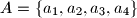$A = \{a_1, a_2, a_3, a_4\}$ of four distinct positive integers, we denote the sum$a_1 +a_2 +a_3 +a_4$ by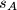$s_A$. Let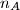$n_A$ denote the number of pairs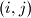$(i, j)$ with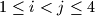$1 \leq i < j \leq 4$ for which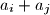$a_i +a_j$ divides$s_A$. Find all sets$A$ of four distinct positive integers which achieve the largest possible value of$n_A$.

Proposed by Fernando Campos, Mexico
Izvor: Međunarodna matematička olimpijada, shortlist 2011